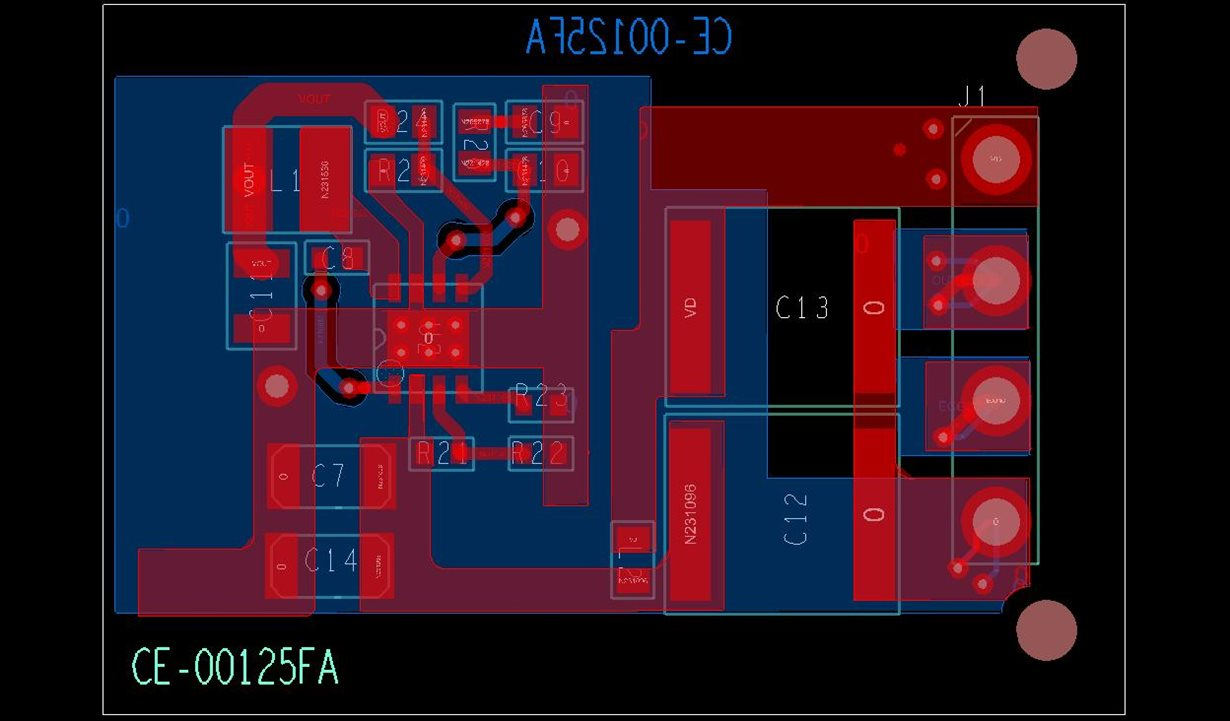If you have a related question, please click the "Ask a related question" button in the top right corner. The newly created question will be automatically linked to this question.

# TPS54061-Q1: If we increase the input voltage, the output ripple will get reduce and waveform get smooth as shown below . Why?

Part Number: TPS54061-Q1

This is regarding TI part “TPS54061-Q1” for automotive application.

We got the Proto-board with this DC-DC converter mounted.

Design specifications

Typical Input Voltage:  24V

Typical Output Voltage:  12V

Typical Load @ 12V: 10-15 mA

DC-DC converter switching Frequency set: Approx 560Khz

During output ripple measurement we have observed the below issue.

1. @24V input the output ripple amplitude is approx 90mV. Should be less then 50 mV

2. The output ripple frequency is approx 115Khz, should be close to DC-DC converter switching frequency.

3. as per attached figure (@24V input), output ripple has two frequency components, The switching frequency(560Khz) is superimposed on lower frequency(115Khz) components.

4. If we increase the input applied voltage to 30V, the output ripple amplitude is approx 50mV and ripple frequency more then 563Khz.

Below is the description of design and measurements

We are using TPS54061-Q1 DC-DC convertor for converting 24V to 12V followed by zener diode as voltage regulator for Vz=6.8V as shown below:

Issue: If we increase the input voltage, the output ripple will get reduce and waveform get smooth as shown below . Why?

Below are the measured ripple with different input voltageFigure 5 Ripple with input 18V Vp-p = 160mv , freq=51KHz Figure 6 Ripple with input 24V Vp-p = 90mv , freq=115KHz Figure 7 Ripple with input 30V Vp-p = 50mv , freq=563KHz Figure 8 Ripple with input 38V Vp-p = 70mv , freq=283KHz

Refer below figure for Layout of design :

Layer in Red is TOP layer and Blue is Bottom layer